Latest Banking jobs   »

# Quantitative Aptitude Quiz For IBPS RRB PO/Clerk Prelims 2022- 19th July

Directions (1-5): Line graph given below shows the selling prices (in rupees) of three types of Refrigerators (A, B & C) in four different years i.e. 2016, 2017, 2018, and 2019 for a shopkeeper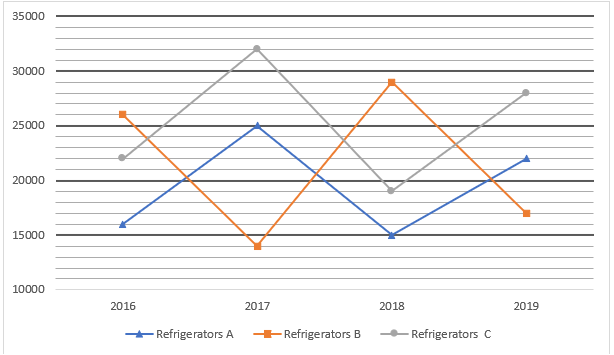Q1. If a discount of 24% is given on refrigerator C sold in 2018 and ratio of MP to CP of C in 2018 is 5 : 3. then find the difference between the discount allowed and profit earned on C in 2018. (in rupees)
(a) 5000
(b) 4000
(c) 2000
(d) 6000
(e) 3000

Q2. Find out average selling price of refrigerator A in all the given years. (in rupees)
(a) 16500
(b) 22500
(c) 18500
(d) 19500
(e) 25500

Q3. Find out the ratio between selling price of refrigerator C in 2018 and the selling price of refrigerator A in 2017?
(a) 20:21
(b) 18:25
(c) 19:25
(d) 23:27
(e) 16:25

Q4. In which year sum of selling price of all 3 type of the refrigerator was the lowest?
(a) 2019
(b) 2016
(c) 2017
(d) 2018
(e) 2016 and 2018

Q5. selling price of refrigerator A in year 2018 is approx. what percent of selling price of refrigerator B in 2019?
(a) 78%
(b) 88%
(c) 82%
(d) 72%
(e) 93%

Directions (6-10): In each of these questions, two equations (I) and (II) are given. You have to solve both the equations and give the answers accordingly.
(a) if x>y
(b) if x≥y
(c) if x<y
(d) if x ≤y
(e) if x = y or no relation can be established between x and y.

Q6. I. x² – 14x + 48 = 0
II. y² – 17y + 72 =0

Q7. I. x² + 13x + 42 = 0
II . y² + 15y + 56 = 0

Q8. I . x² + 8x + 12 = 0
II . 6y² + 13y + 6 = 0

Q9. 2x² + 9x + 9 = 0
y² + 28y + 192 = 0

Q10. I . x² – 9x + 20 = 0
II. y² – 6y + 9 = 0

Directions (11-15): What should come in place of the question mark (?) in the following number series.

Q11. 90, 55, 75, 142.5, ? , 862.5
(a) 285
(b) 325
(c) 470
(d) 855
(e) 270

Q12. 5, 12, 39, 160, ? , 4836
(a) 850
(b) 750
(c) 800
(d) 805
(e) 820

Q13. 26, 36, 54, 80, 114, ?
(a) 146
(b) 133
(c) 201
(d) 134
(e) 156

Q14. 17, 25, 49, 97, 177, ?
(a) 297
(b) 247
(c) 358
(d) 292
(e) 279

Q15. 21, 28, 42, 64, 95, ?
(a) 125
(b) 158
(c) 142
(d) 136
(e) 164

Solutions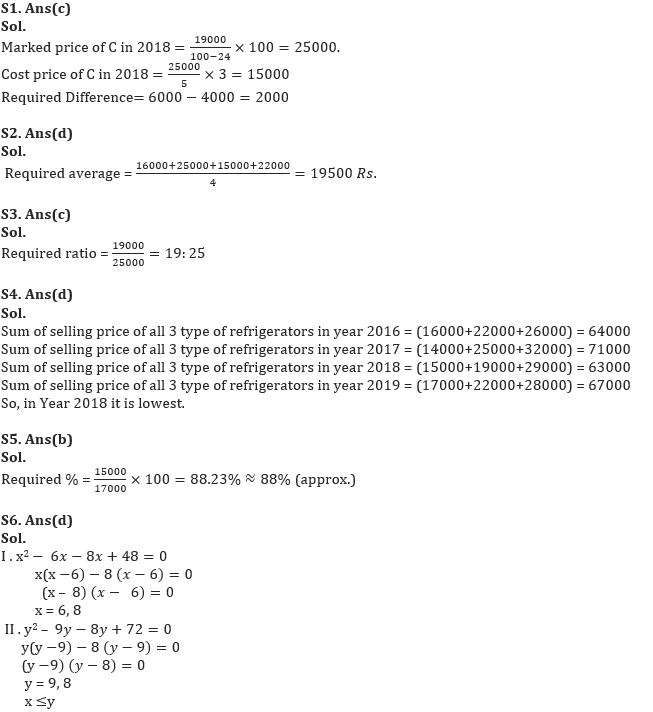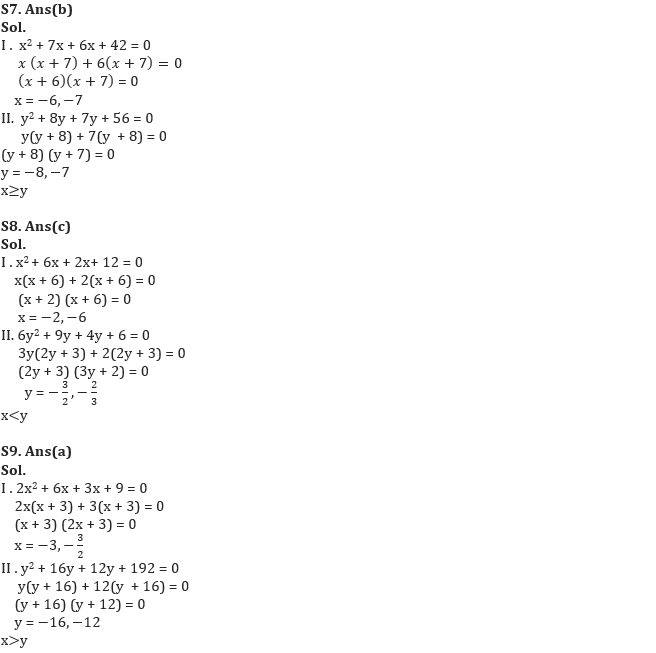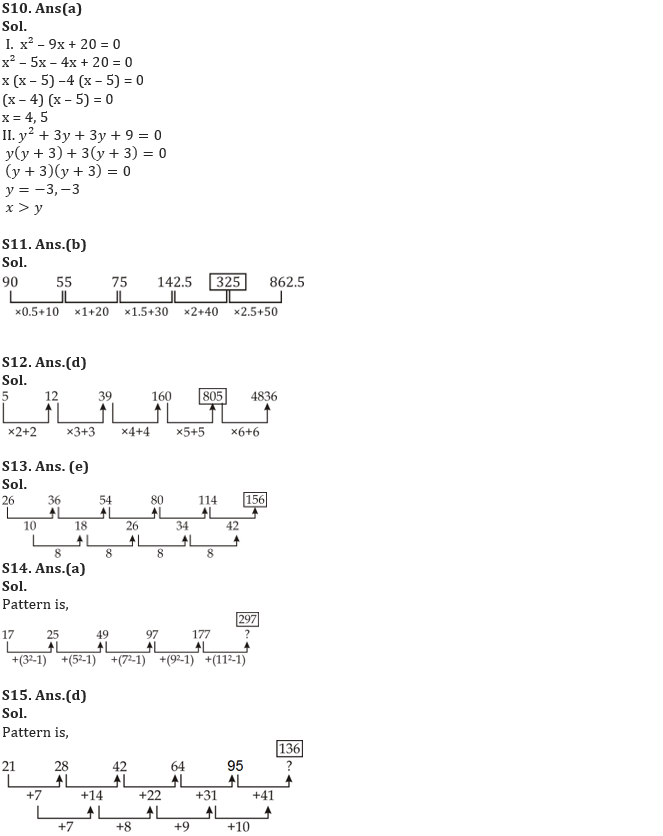#### Congratulations!Incorrect details? Fill the form again here

•Reasoning Ability Quiz For IBPS RRB PO C...
•Quantitative Aptitude Quiz For IBPS RRB ...
•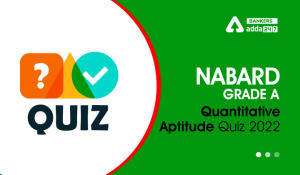Quantitative Aptitude Quiz For NABARD Gr...
•Reasoning Ability Quiz For IBPS RRB PO C...
•Quantitative Aptitude Quiz For IBPS RRB ...
•Quantitative Aptitude Quiz For NABARD Gr...